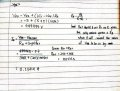# DC Analysis of BJT

#### DCJW

Joined May 18, 2022
11

#### LvW

Joined Jun 13, 2013
1,466
Please, don`t use symbols (like VBB etc) not shown in the circuit.
More than that, explain the formula mentioned in your text.
Otherwise, we cannot comment.

#### DCJW

Joined May 18, 2022
11
VBB is the base voltage of a bipolar junction transistor, which can be calculated using the formula above which is VBB=VEE+(VCC-VEE)RB then only use these values to calculate the value of IB which is the base current, but then after I found the value of IB there will be a big difference compared to when I do a simulation in LTSpice.

VEE=-5
VCC=+5
β=200
VBE(on)=0.7#### LvW

Joined Jun 13, 2013
1,466
Referring to the first (handwritten) formula:
Did you realize that at the right side of the equation we have "voltage*Ohm=voltage"?

#### DCJW

Joined May 18, 2022
11
Do you know how to write a KVL so be able to solve for Vb in this circuit:
View attachment 270118
Thanks, I had successfully solved the DC analysis of this circuit, thru your advice given I am able to solve the dc analysis.

#### DCJW

Joined May 18, 2022
11
Referring to the first (handwritten) formula:
Did you realize that at the right side of the equation we have "voltage*Ohm=voltage"?
Ya, I now only realize the problem in this formula, Thanks for your advice.

#### Jony130

Joined Feb 17, 2009
5,351
So for this case the emitter current is Ie = (5V - 0.7V)/(100kΩ/(200 + 1) + 240Ω + 20kΩ) ≈ 0.2074mA ≈ 207.4μA Thus, the base current is 1.032μA which means that the base voltage will be very close to 0V (-103mV).

•DCJW